# Info on exponential approaches

### As told by Carlos Jambrina on 19/02/2019

A common pattern in gamedev is to interpolate a value towards a given target over time, with an update speed that depends on the difference between the two values:

`current = current + (target - current) * expFactor`

The result is actually quite snappy and smooth: the value covers large deltas quickly, then slows down for small adjustments. It's responsive to sudden changes, and prevents jittering at the same time.

A sample update from 0 to 1 with expFactor = 0.25 would look like this: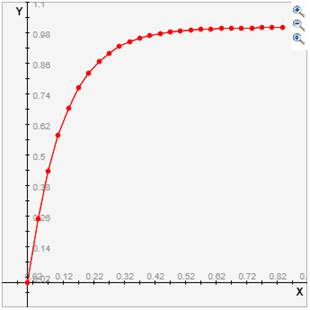There's a property of this method, however, that will likely lead to issues: the rate at which our value approaches the target depends on the number of times the equation gets called, and is independent of the actual amount of time passed. Calling this code 30 times a second will result in a different behavior than if your update rate is 60.

Consider an entity that's constantly turning, and every update we approach the current direction to a value 90 degrees to the right, using this same equation. Different frame update rates will lead to different turn rates: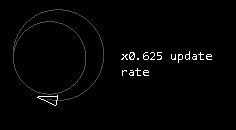There's a way around this though, if we consider exponential functions. Let's take a look at 1ekxFor x = 0, the function returns 1, and by x ≈ 7 the value will pretty much be equal to zero.

If we consider the x axis to be seconds, we can use k as a tunable factor to expand or contract the curve. Let's refer to k as 1timeHorizon. Larger timeHorizon values will lead to larger convergence times, and viceversa: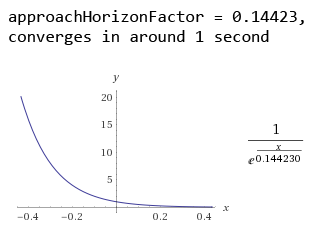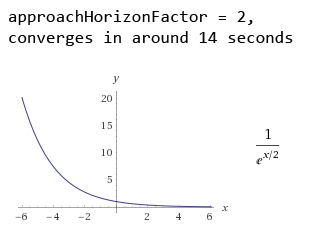Since x is seconds, we can pass in the time delta from our update, and we'll now have a time-dependent update curve. Note that 1ekx goes from 1 to 0, which is why our delta goes from target to current:

`current = target + (current – target) / e(dt/timeHorizon)`

Playing around with values for timeHorizon, we can reach a shape similar to the one we started with:So far we've been using just pure intuition, but we can actually proof that this equation represents the same curve we started with. This posts explains it very nicely:

https://gamedev.stackexchange.com/questions/80089/how-to-use-weighted-average-easing-correctly-with-variable-framerate/80121#80121

Some nice conclusions from this:

• Our resulting function (let's call it expApproach) is time (not frame) dependent, and doesn't change its output when called at different frequencies: expApproach((expApproach(value, target, time1), target, time2) = expApproach(value, target, time1 + time2).
• Since our timedeltas will pressumably be small, we can approximate e via Taylor with a low number of terms, and the error won't be too significant.
• We now have an analytical way of mapping expFactor to timeHorizon.

For the previous case of the entity that wanted to turn right, we can now see how the suggested equation solves our issues with varying time rates: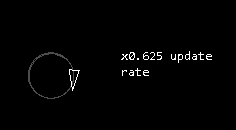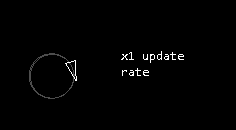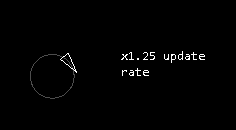This is all great, but it's still not entirely trivial how to map existing behaviors using the initial function to the timeHorizon suggested here. I set up a tool to maybe help with that:

Exponential approach tool

I'm hoping it's self explanatory, but just in case, here's how it looks when trying to replicate the curve we started with, with an expFactor of 0.25, tuned for a game that runs at 30 frames per second:We got a match with timeHorizon = 0.116!

So, TLDR:

Whenever you have issues using:

`current = current + (target - current) * expFactor`

`current = target + (current – target) / e(dt/timeHorizon)`